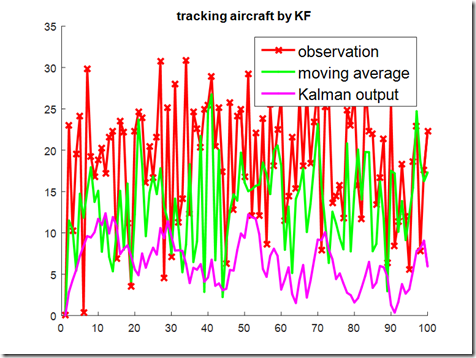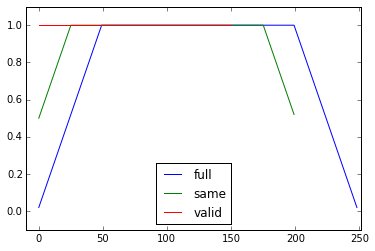[开发技巧]·Python极简实现滑动平均滤波（基于Numpy.convolve）3.Numpy.convolve介绍

numpy.convolve(a, v, mode=‘full’)

a:(N,)输入的一维数组
v:(M,)输入的第二个一维数组
mode:{‘full’, ‘valid’, ‘same’}参数可选
‘full’　默认值，返回每一个卷积值，长度是N+M-1,在卷积的边缘处，信号不重叠，存在边际效应。
‘same’　返回的数组长度为max(M, N),边际效应依旧存在。
‘valid’ 　返回的数组长度为max(M,N)-min(M,N)+1,此时返回的是完全重叠的点。边缘的点无效。

4.算法实现

def np_move_avg(a,n,mode="same"):
return(np.convolve(a, np.ones((n,))/n, mode=mode))mode的参数np.convolve指定如何处理边缘。在这里选择了same模式，这样可以保证输出长度一种，但你可能还有其他优先事项。这是一个说明模式之间差异的图：

import numpy as np
import matplotlib.pyplot as plt

def np_move_avg(a,n,mode="same"):
return(np.convolve(a, np.ones((n,))/n, mode=mode))

modes = ['full', 'same', 'valid']
for m in modes:
plt.plot(np_move_avg(np.ones((200,)), 50, mode=m));

plt.axis([-10, 251, -.1, 1.1]);

plt.legend(modes, loc='lower center');

plt.show()5.参考

posted @ 2019-05-16 11:35  小宋是呢  阅读(16568)  评论(0编辑  收藏  举报